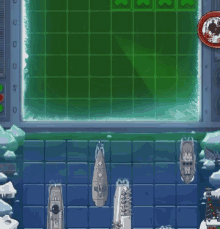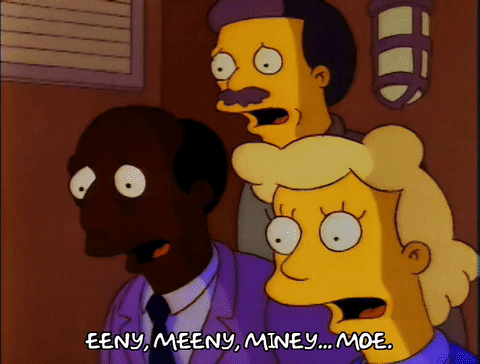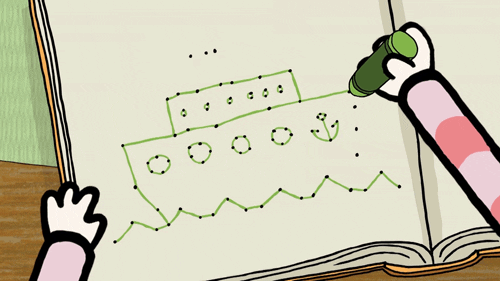# Battleship

## Create a Battleship guessing game with C#!@JakeGerberIn this workshop, we're going to make a fully-functional implementation of the popular game Battleship, in C#! Ready to get started?Final Demo and Code

## Getting started

We're going to use Repl.it, a free, online code editor, to create the project. To get started, visit repl.it/languages/c#.Don't worry if you've never written C# before. As long as you've written code in most languages before, you'll be able to easily pick it up!

## Initializing the BoardWhen your repl spins up, you should see this code already added in the `main.cs` file:

``````using System;

class MainClass {
static void Main(string[] args)
{
}
}
``````

Let's start by creating two 2D arrays of characters.

``````static void Main(string[] args)
{
char[,] actualBoard = new char[6, 6];
char[,] board = new char[6, 6];
}
``````

`actualBoard` refers to the answer key, and `board` refers to the user guessing board.

Next, create two for loops, one nested within the other, that loops through each square of the board.

``````static void Main(string[] args)
{
//Previous code would be here.
for (int i = 0; i < board.GetLength(0); i++)
{
for (int x = 0; x < board.GetLength(1); x++)
{
board[i, x] = '.';
actualBoard[i, x] = '.';
}
}
}
``````

Inside these loops, we set the value of every piece of both boards to a dot. This represents an empty space (like the water in Battleship).

Next, after both for loops complete, create a new Random object.

``````static void Main(string[] args)
{
//Previous code would be here.
Random random = new Random();
}
``````

Random is a library built in to C# that lets us easily generate random values. Soon, we're going to use this for the random ship placement.

## Ship PlacementTime to place the ship on the board. But where is the ship going to be placed? Well, this is where the `Random` object we created earlier comes in handy. Under all of the code you just wrote, but still in the `Main` function, add:

``````static void Main(string[] args)
{
// The code we already wrote would be here.

//horizontal vs vertical
int dir = random.Next(0, 2);
}
``````

Here, we're initializing an integer called `dir` that generates a random that's either 1 or 2. (The upper bound of a Random object is not included, so if we want to choose between 0 and 1, the upper limit needs to be 2). This will represent whether the ship will be vertical or horizontal.

### Horizontal placement

Let's write some code that handles the random value we just generated.

``````static void Main(string[] args)
{
// The code we already wrote would be here.

// horizontal ship
if (dir == 0)
{
int x = random.Next(0, 3);
int y = random.Next(0, 6);

actualBoard[x, y] = 'X';
actualBoard[x + 1, y] = 'X';
actualBoard[x + 2, y] = 'X';
}
}
``````
• If `dir` equals 0, then the ship will be placed horizontally.
• We generate two random numbres to represent a random place on the board.
• We set the random coordinates on the `actualBoard` to `X`, to represent the placement of a ship.
• Then, we also set 2 additional x coordinates to an `X`, to give the ship some length.
• The randomness specifies that it will stay within the bounds of the array so it will not cause an error.

### Vertical

Now, let's handle vertical placement. Add an `else if` statement that checks if `dir` equals 1.

``````static void Main(string[] args)
{
// The code we already wrote would be here.

//horizontal ship
if (dir == 0)
{
//Code we just wrote.
}
//vertical ship
else if (dir == 1)
{
int x = random.Next(0, 6);
int y = random.Next(0, 3);

actualBoard[x, y] = 'X';
actualBoard[x, y + 1] = 'X';
actualBoard[x, y + 2] = 'X';
}
}
``````
• If `dir` equals 1, then the ship will be placed vertically.
• Add the same code you added for the horizontal placement, except switch the random bounds for the x and y coordinates and increment 2 `y` values instead of 2 `x` values.

### Ending Statements

``````static void Main(string[] args)
{
// The code we already wrote would be here.

int shipPieces = 3;
int shipHits = 0;
int guesses = 0;
}
``````

Here, we're creating 3 integer variables to track the ship pieces, the ship hits, and the guesses. We'll use these when the user starts guessing.

## GuessingGuessing is the main part of this game. So, let's add that functionality!

Under the code you just wrote, at the bottom of the `Main` method, add an infinite `while` loop:

``````static void Main(string[] args)
{

while (true)
{
}
}
``````

Inside the `while` loop, add a `try-catch` block:

``````while (true)
{
try
{
}
catch
{
}
}
``````

This try-catch block will catch and bad inputs that cause errors. If we don't include this, then our program will break if a user enters something invalid!

### User Input

Inside the `try` block, let's add a way for the program to accept user input and make a guess.

Start by calling the `drawBoard()` function. We haven't written this function yet, but we will in a bit, so don't worry :)

``````try
{
drawBoard(board);
}
``````

``````try
{
drawBoard(board);
Console.Write("Enter a letter: ");
colLetter = colLetter.ToUpper();
int row = 0;
}
``````

This code:

• Prompts the user for input
• Uses `Console.ReadLine()` to accept user input
• Converts their letter to uppercase
• Initializes an intege variable called `row` and sets it to 0. We'll use this variable in a moment.

Next, add the following 3 lines:

``````try
{
drawBoard(board);
Console.Write("Enter a letter: ");
colLetter = colLetter.ToUpper();
int row = 0;

Console.Write("Enter a number: ");
int col = Int32.Parse(rowInput)-1;
}
``````

These lines:

• Prompt the user for a number
• Accept their input
• Parse the integer (by default, console input is a string, so we want to convert it to an integer type)
• Subtract 1 so that its placement on the board can be more accurate. (The first index of an array is 0, so if we didn't do this, the user's guess would be 1 off)

### Letter Input to Number

At first, we asked the user for a letter. But we can't input a letter into our 2D array. So, we'll need to convert the letters to row numbers.

At the bottom of the `try` block, add:

``````try
{
//What we just wrote is here.

if (colLetter == "A")
{
row = 0;
}
else if (colLetter == "B")
{
row = 1;
}
else if (colLetter == "C")
{
row = 2;
}
else if (colLetter == "D")
{
row = 3;
}
else if (colLetter == "E")
{
row = 4;
}
else if (colLetter == "F")
{
row = 5;
}
}
``````

Now, rows A-F are converted to rows 1-5.

### Checking Guess

Was the user's guess correct? Let's write some code to find out.

``````try
{
//Previous code would be here.
if (board[row, col] == '.')
{
guesses++;
if (actualBoard[row, col] == 'X')
{
board[row, col] = 'X';
shipHits++;
Console.WriteLine("Hit!");
}
else
{
board[row, col] = 'O';
Console.WriteLine("Miss!");
}
}
}
``````
• This if statement makes sure the spot that the user guessed has not been guessed before (if it hasn't been guessed before, the spot will be a `.`.
• First, we increment the number of guesses by 1.
• Then, we write an if/else statement to check if their guess is a hit on the answer key board.
• If it's a hit, we set that spot on the user's board to `X` to indicate a hit. Then we tell the user they've hit.
• If it's a miss, we set that spot on the user's board to `0` to indicate a miss. Then we tell the user they've missed.

Next, inside the if statement that checks if it's a hit, add this second if statement, which compares the `shipHits` and `shipPieces` integers. If they're equal, then the user has guessed all of the pieces of ship, so we break out of the outer `while (true)` loop.

``````if (actualBoard[row, col] == 'X')
{
board[row, col] = 'X';
//Console.WriteLine("Hit!");
shipHits++;
if (shipHits == shipPieces)
{
break;
}
}
``````

### Ending Statements

Finally, we call the `drawBoard()` function again to draw the final board, and we let the user know that they won the game.

``````while (true)
{
}
drawBoard(board);
Console.WriteLine(\$"You won with {guesses} guesses!");
``````

The program won't break out of the loop until the user wins the game, so we know that if they reach that `Console.WriteLine` statement, they've won.

## Drawing the BoardNow, let's write the long-awaited `drawBoard()` method!

### Creating the Function

Inside of your `MainClass`, but after the `Main` method, create a new function called `drawBoard()`, which accepts a 2D array of characters, like so:

``````using System;

class MainClass {
static void Main(string[] args)
{
}

public static void drawBoard(char[,] arr)
{
}
}
``````

### Top Numbers

Inside the newly-created `drawBoard()` method, add this code:

``````public static void drawBoard(char[,] arr)
{
Console.Clear();
int asciiVal = 65;
}
``````

This code:

• Clears the console so we can redraw the board.
• Creates an integer value that's set to 65, the ASCII value for "A".

``````public static void drawBoard(char[,] arr)
{
Console.Clear();
int asciiVal = 65;

Console.Write(" \t");
for (int i = 1; i < arr.GetLength(1) + 1; i++)
{
Console.Write(\$"{i}\t");
}
Console.WriteLine("\n");
}
``````

Here, we're just drawing the top row of numbers (1-6). This is mainly a lot of spacing. Don't look too deep into it. `\t` creates a tab, and `\n` creates a new line.

### Drawing the Board

Let's write the rest of the method for drawing the board. Inside the `drawBoard()` method, add:

``````public static void drawBoard(char[,] arr)
{
// code we just wrote

for (int i = 0; i < arr.GetLength(0); i++)
{
Console.Write(\$"{(char)asciiVal}\t");
for (int x = 0; x < arr.GetLength(1); x++)
{
Console.Write(\$"{arr[i, x]}\t");
}
Console.WriteLine();
asciiVal++;
}
}
``````

Here, we:

• Loop through the board that was passed in
• Casts the ASCII value we set earlier to a character, and prints it, along with a tab at the end
• Loops through the rest of the board 2D array
• Prints the value of each square (remember, either `.`, `X`, or `0`), along with a tab at the end
• Prints an empty line (for readability)
• Increments the ASCII value so that it increments the rows from A, to B, to C, etc. (if this doesn't make sense, you can see it for yourself in a moment)

## You're done!I sunk your battleship! Wait, wrong line. I mean you've finished the workshop! That sounds better.

Try it yourself! Play the game with the computer by clicking the green "Run" button at the top of your repl.

Final code:
``````using System;

class MainClass {
static void Main(string[] args)
{
//1 person battleship game
char[,] actualBoard = new char[6, 6];
char[,] board = new char[6, 6];

for (int i = 0; i < board.GetLength(0); i++)
{
for (int x = 0; x < board.GetLength(1); x++)
{
board[i, x] = '.';
actualBoard[i, x] = '.';
}
}

Random random = new Random();

//horizontal vs vertical
int dir = random.Next(0, 2);

//vertical ship
if (dir == 0)
{
int x = random.Next(0, 3);
int y = random.Next(0, 6);

actualBoard[x, y] = 'X';
actualBoard[x + 1, y] = 'X';
actualBoard[x + 2, y] = 'X';

}
//horizontal ship
else if (dir == 1)
{
int x = random.Next(0, 6);
int y = random.Next(0, 3);

actualBoard[x, y] = 'X';
actualBoard[x, y + 1] = 'X';
actualBoard[x, y + 2] = 'X';
}

int shipPieces = 3;
int shipHits = 0;
int guesses = 0;

while (true)
{
try
{
drawBoard(board);
Console.Write("Enter a letter: ");
colLetter = colLetter.ToUpper();
int row = 0;

Console.Write("Enter a number: ");
int col = Int32.Parse(rowInput)-1;

if (colLetter == "A")
{
row = 0;
}
else if (colLetter == "B")
{
row = 1;
}
else if (colLetter == "C")
{
row = 2;
}
else if (colLetter == "D")
{
row = 3;
}
else if (colLetter == "E")
{
row = 4;
}
else if (colLetter == "F")
{
row = 5;
}

if (board[row, col] == '.')
{
guesses++;
if (actualBoard[row, col] == 'X')
{
board[row, col] = 'X';
//Console.WriteLine("Hit!");
shipHits++;
if (shipHits == shipPieces)
{
break;
}
}
else
{
board[row, col] = 'O';
//Console.WriteLine("Miss!");
}
}
}
catch
{
}

}

drawBoard(board);
Console.WriteLine(\$"You won with {guesses} guesses!");

}

public static void drawBoard(char[,] arr)
{
Console.Clear();
int asciiVal = 65;

Console.Write(" \t");
for (int i = 1; i < arr.GetLength(1) + 1; i++)
{
Console.Write(\$"{i}\t");
}
Console.WriteLine("\n");

for (int i = 0; i < arr.GetLength(0); i++)
{
Console.Write(\$"{(char)asciiVal}\t");
for (int x = 0; x < arr.GetLength(1); x++)
{
Console.Write(\$"{arr[i, x]}\t");
}
Console.WriteLine();
asciiVal++;
}
}
}
``````

## Hacking

The fun doesn't stop here! Here are some ways you can expand on this project:

Happy hacking!